Ex 7.3

Chapter 7 Class 6 Fractions
Serial order wise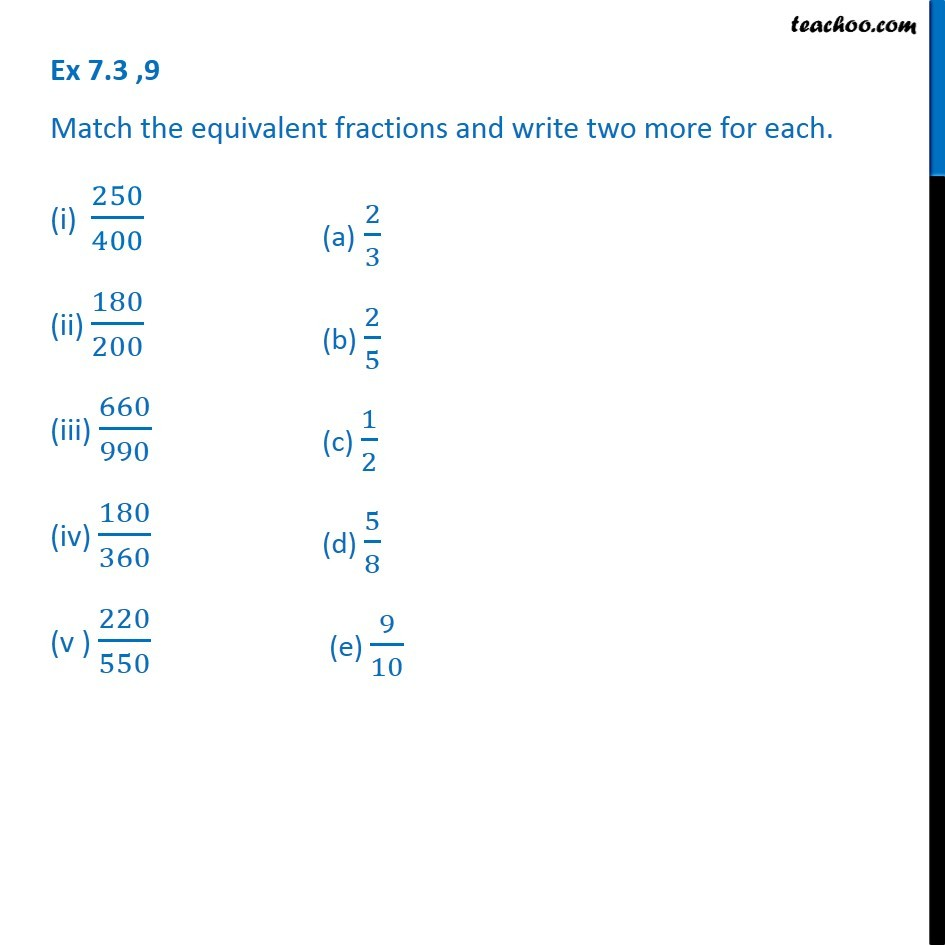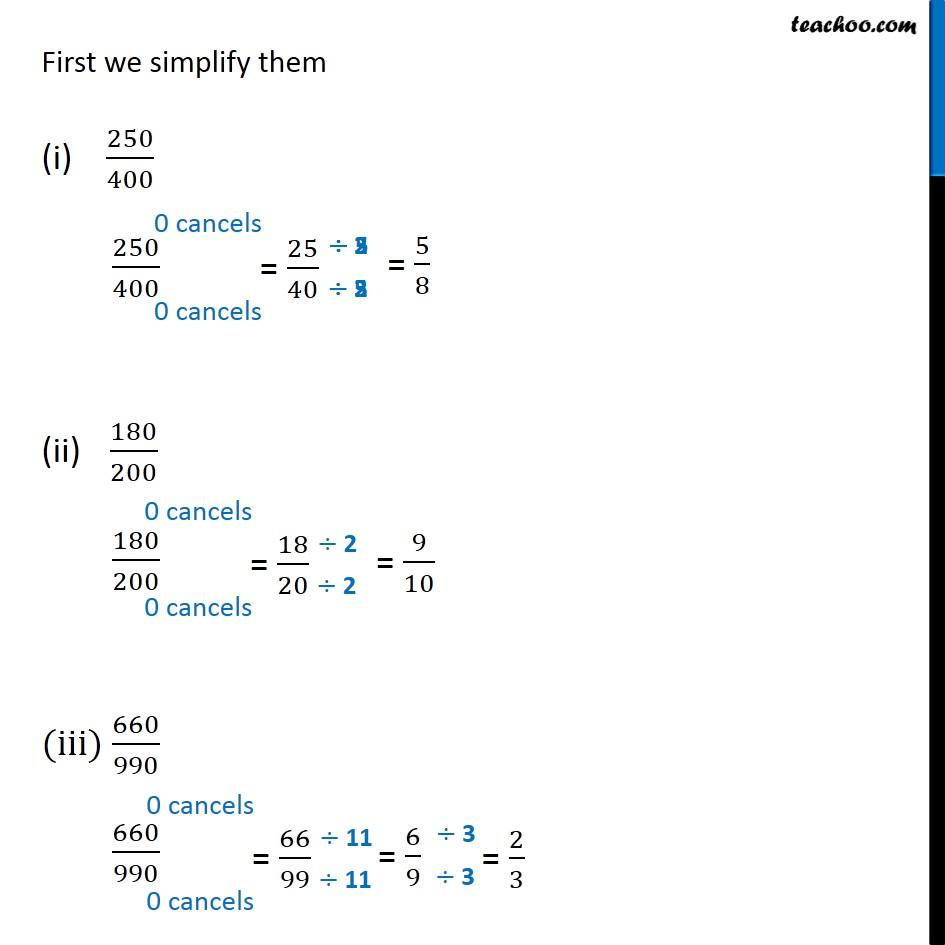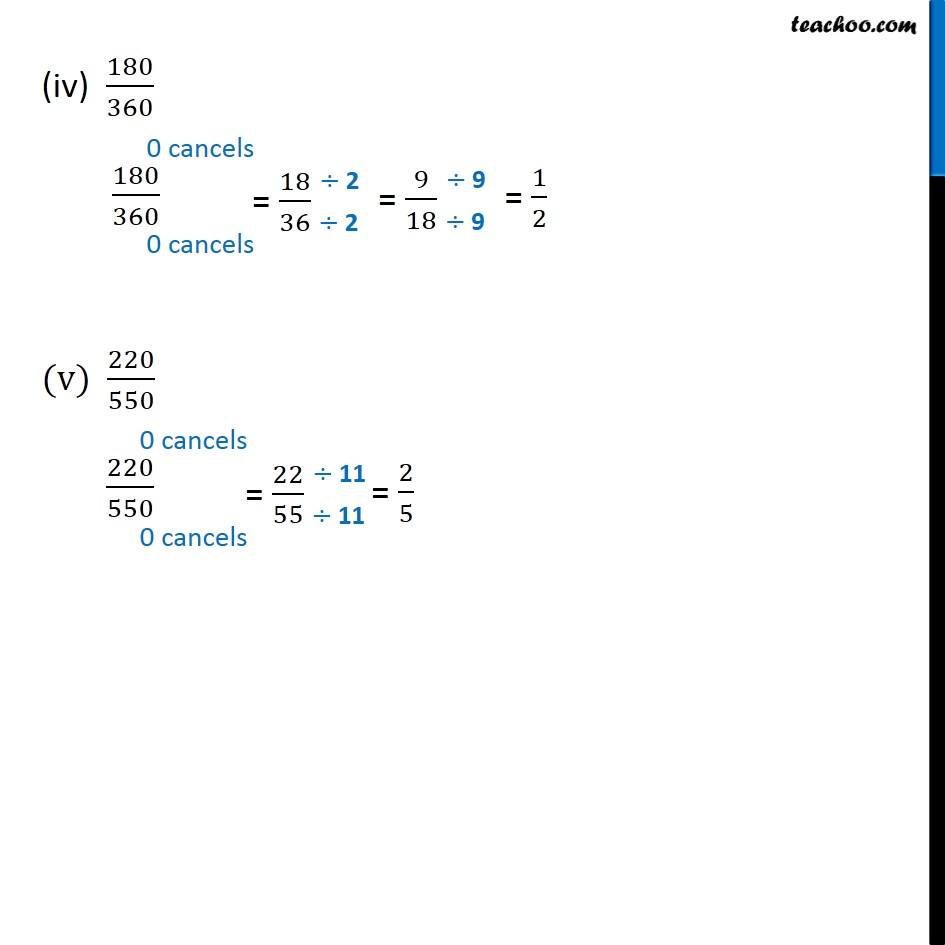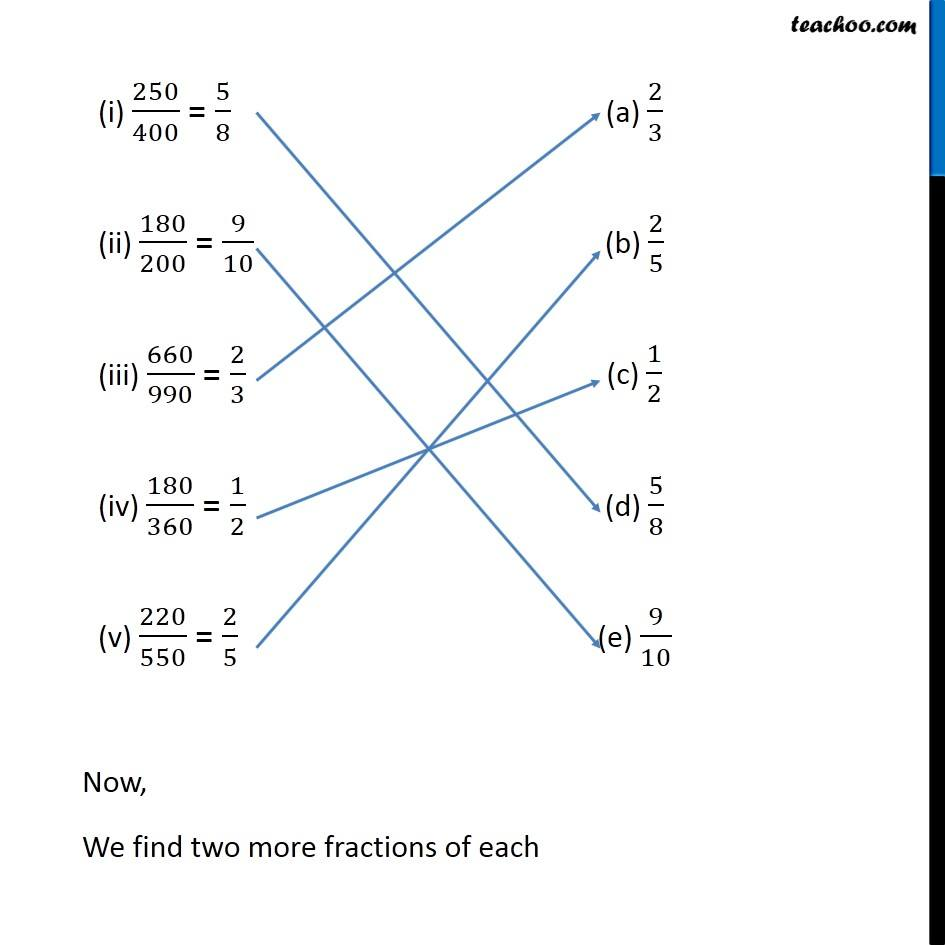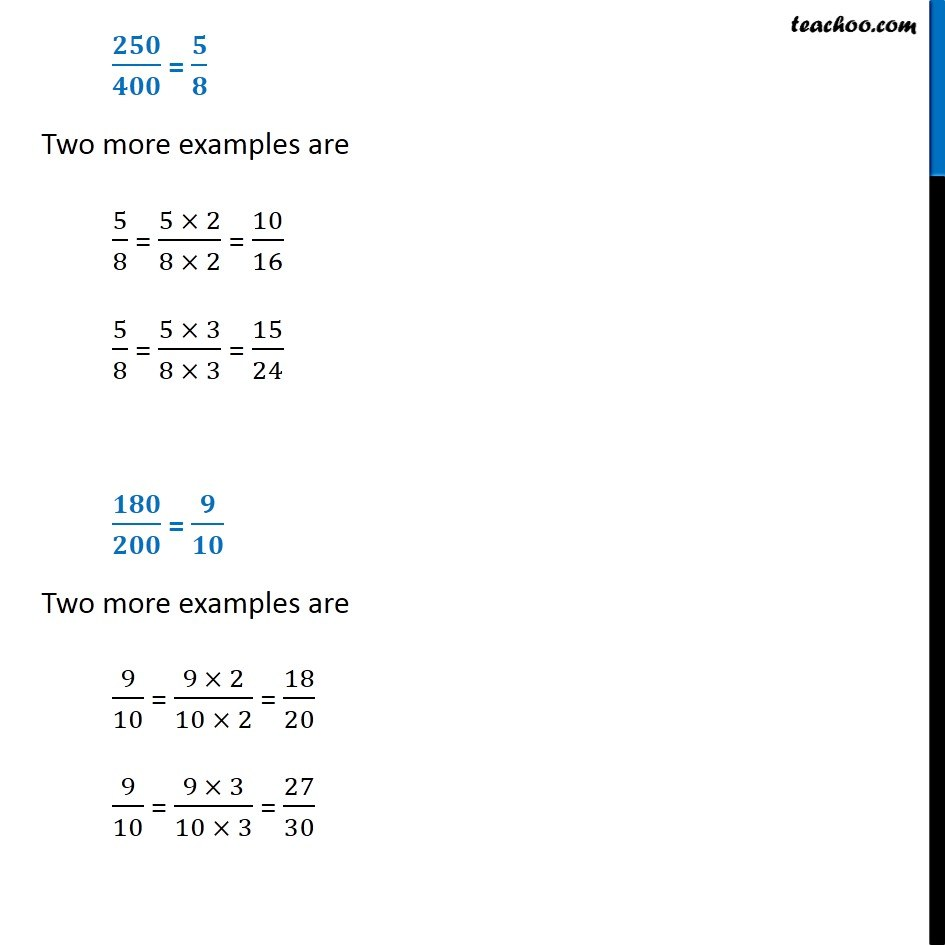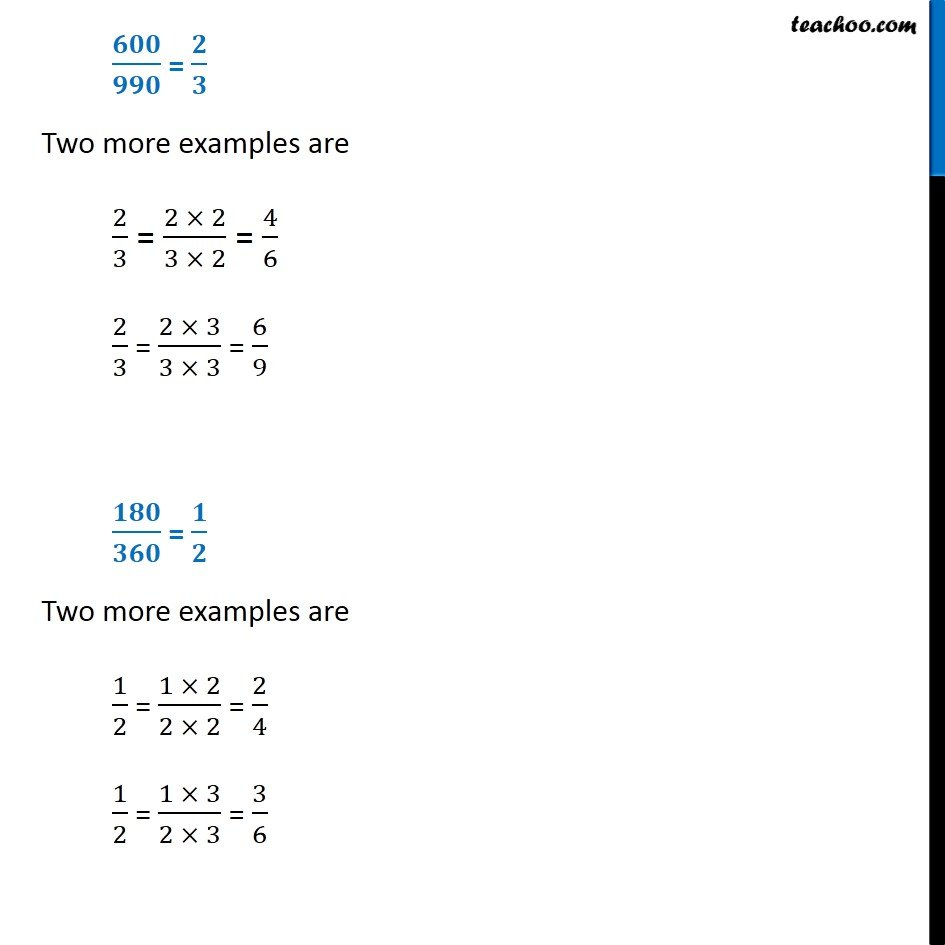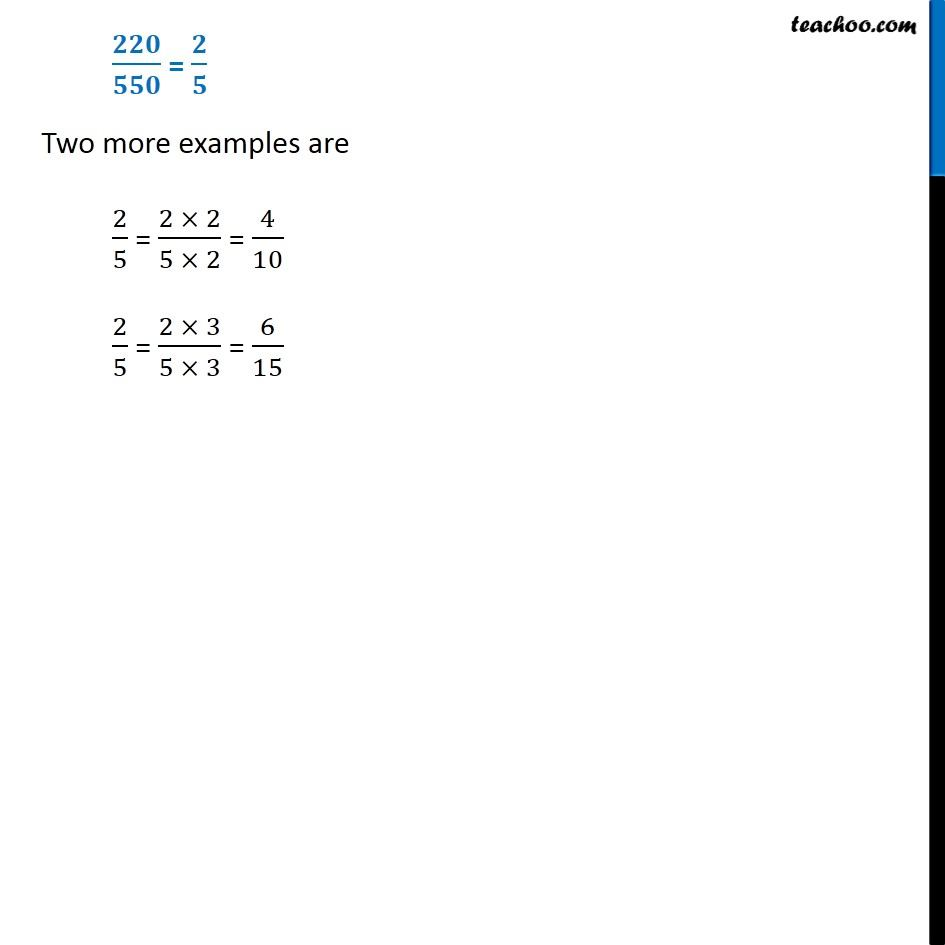Learn in your speed, with individual attention - Teachoo Maths 1-on-1 Class

### Transcript

Ex 7.3 ,9 Match the equivalent fractions and write two more for each. (i) 250/400 (ii) 180/200 (iii) 660/990 (iv) 180/360 (v ) 220/550 (a) 2/3 (b) 2/5 (c) 1/2 (d) 5/8 (e) 9/10 First we simplify them (i) 250/400 250/400 180/200 180/200 (iii) 660/990 660/990 (iv) 180/360 180/360 (v) 220/550 220/550 Now, We find two more fractions of each 𝟐𝟓𝟎/𝟒𝟎𝟎 = 𝟓/𝟖 Two more examples are 5/8 = (5 × 2)/(8 × 2) = 10/16 5/8 = (5 × 3)/(8 × 3) = 15/24 𝟏𝟖𝟎/𝟐𝟎𝟎 = 𝟗/𝟏𝟎 Two more examples are 9/10 = (9 × 2)/(10 × 2) = 18/20 9/10 = (9 × 3)/(10 × 3) = 27/30 𝟔𝟎𝟎/𝟗𝟗𝟎 = 𝟐/𝟑 Two more examples are 2/3 = (2 × 2)/(3 × 2) = 4/6 2/3 = (2 × 3)/(3 × 3) = 6/9 𝟏𝟖𝟎/𝟑𝟔𝟎 = 𝟏/𝟐 Two more examples are 1/2 = (1 × 2)/(2 × 2) = 2/4 1/2 = (1 × 3)/(2 × 3) = 3/6 𝟐𝟐𝟎/𝟓𝟓𝟎 = 𝟐/𝟓 Two more examples are 2/5 = (2 × 2)/(5 × 2) = 4/10 2/5 = (2 × 3)/(5 × 3) = 6/15